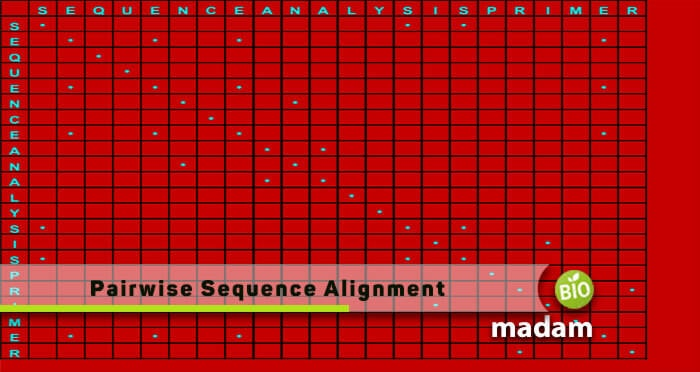# Pairwise Sequence AlignmentPairwise Sequence Alignment is a common method of identification of regions of similarity. These areas usually examine the structural, functional, or evolutionary connections between the two proteins or nucleic acids. This advanced methodology is also helpful for non-biological sequences, for instance, analyzing the distance costs or differences in financial data.

## Representation

We commonly represent the aligned sequence in graphical or text format. Moreover, the amino acid or nucleotide sequencing is represented in rows within a matrix. For the text formats, conservation symbols are used to identify the aligned columns within a matrix. There are particular gaps adjusted between the residues for successive columns.

## Types of Pairwise Sequence Alignment

There are typically two types of alignments, such as

• Local Alignment
• Global Alignment

### Local Alignment

It is the sequencing of two sub-divisions for a couple of sequences. Such an alignment perfectly matches the genomic DNA that has local similarity regions inserted in a non-homologous sequence.

### Global Alignment

It is the sequencing of an entire nucleic acid or protein arrangement length. Global alignment is thought to have sequencing lengths of homologous nature.

## Example

We suppose two sequencings for pairwise sequence alignment, which are as follow:

Sequence A: CGGATCAT

Sequence B: CTTAACT

After aligning A and B:

CGGATCA – – T sequence A

C – – – TTAACT sequence B

## Methods of Pairwise Sequence Alignment

There are several alignment tools present online, but generally, we have three methods of pairwise sequencings, such as:

• Dot-matrix Analysis
• Dynamic Programming
• Word or K-Tuple method

Let’s discuss the three of them, one by one.

### Dot-Matrix Analysis

This method of sequencing represents the similarities between the two closely related sequences. Dot-matrix analysis has two sequences, named A and B. Sequence A comes on top of the matrix, while sequence B is written vertically on the left of the matrix. Start checking from sequence B and see the characters of A. Observe where the characters of A and B match, and insert a dot there. Continue putting the dots this way until it provides a diagonal row of dots.

Note: The dots other than this diagonal line are just random matches.

#### Dot-Matrix Comparison

The diagonal matrix tells us the similarities between the two closely related nucleotide sequences. The word “similarities” means there is no nucleotide character matching in both sequences. Instead, the similarities indicate the relationship between organisms and their ancestors. It helps us examine how similar they are in their functioning and structure.

Dot-Matrix shows the insertion or deletion of sequences to let us know about mutations. If in case, a mutation occurs, the diagonal will shift. Furthermore, it’s pretty impossible to tell if the shifted diagonal is due to insertion or deletion, so we pronounce it as “indels.”

This pairwise sequencing method is highly known to represent “palindromic sequences.” It means the arrangement remains the same, even if we read it from left to right or right to left. We can observe such a sequence if there are some perpendicular diagonals at the original diagonal.

Another prediction by this comparison is gene duplication. It shows a parallel diagonal within the matrix.

Everything comes with its pros & cons, so does the dot-matrix method.

The position of dots tells us about the region of alignment. It gives all possible alignments or diagonals. Experts mostly use the human brain and eyes in this technique.

The major disadvantage of this method is that it does not give us optimal alignment.

### Dynamic Programming Method

This type of sequencing is used to obtain an optimal alignment. The dynamic programming method tells us about gaps that could be a mutation. These are typically represented by “—.” Experts apply this technique to generate local alignments via the Smith-Waterman algorithm, while global alignments are through the Needleman-Wunsch algorithm. Both the processes have a slight difference.

#### Smith-Waterman Algorithm

The Smith-Waterman algorithm utilizes four values, with one being zero. This zero benefits as it can be replaced with any negative number in the matrix. The S-W technique is advantageous as we can move the maximum value present in the matrix to the top left corner.

#### Needleman-Wunsch Algorithm

In contrast, this technique of global alignment uses only three values instead of four. These are:

• The first one as the diagonal value
• The second for match/miss-match
• The third is as a gap penalty

This method is well-known for finding an optimal alignment through the scoring function. It helps align the nucleotide to protein sequences.

This type is limited to only two or a few sequences aligning. The method turns slow for extremely long nucleotide sequences.

### Word/K-Tuple Method

It is the heuristic method, which means it will not provide an extremely efficient result, but is significant to get immediate outputs. Word method is also called the K-Tuple method, which is better than dynamic programming. It is preferable as it works for large-scale database sequencing. The word K-Tuple means a string of K words.

For Example

For nucleotide K = 11 and protein K = 3

K method is implemented in the FASTA and BLAST families. In FASTA, the specific length of words = k is defined by the user to search a database. It is not so fast, but it is susceptible at a low value of k. In BLAST, algorithms are used for specific queries and match distantly related sequences.

## Final Words

Despite using any method of pairwise sequence alignment, the purpose will always remain the same. It’s just that with the advancements, the methodologies also progress with lesser chances of errors. Till now, the word method is efficient of all three methods discussed above.